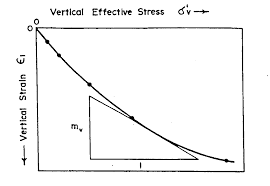## How to Calculate and Solve for Vertical Strain | Rock MechanicsThe image above represents vertical strain.

To compute for vertical strain, five essential parameters are needed and these parameters are Vertical Stress (σv), Poisson’s Ratio (v), Principal Horizontal Stress Component 1 (σH1), Principal Horizontal Stress Component 2 (σH2) and Young’s Modulus (E).

The formula for calculating the vertical strain:

εv = v – vσH1 – vσH2) / E

Where:

εv = Vertical Strain
σv = Vertical Stress
v = Poisson’s Ratio
σH1 = Principal Horizontal Stress Component 1
σH2 = Principal Horizontal Stress Component 2
E = Young’s Modulus

Let’s solve an example;
Find the vertical strain with a vertical stress of 44, Poisson’s ratio of 5, principal horizontal stress component 1 of 6, principal horizontal stress component 2 of 4 and young’s modulus of 12.

This implies that;

σv = Vertical Stress = 44
v = Poisson’s Ratio = 5
σH1 = Principal Horizontal Stress Component 1 = 6
σH2 = Principal Horizontal Stress Component 2 = 4
E = Young’s Modulus = 12

εv = v – vσH1 – vσH2) / E
εv = (44 – 5(6) – 5(4)) / 12
εv = (44 – 30 – 20) / 12
εv = -6 / 12
εv = -0.5

Therefore, the vertical strain is -0.5.

Calculating the Vertical Stress when the Vertical Strain, Poisson’s Ratio, Principal Horizontal Stress Component 1, Principal Horizontal Stress Component 2 and Young Modulus is Given.

σv = (εv x E) + vσH1 + vσH2

Where:

σv = Vertical Stress
εv = Vertical Strain
v = Poisson’s Ratio
σH1 = Principal Horizontal Stress Component 1
σH2 = Principal Horizontal Stress Component 2
E = Young’s Modulus

Let’s solve an example;
Find the vertical stress with a vertical strain of 52, Poisson’s ratio of 12, principal horizontal stress component 1 of 15, principal horizontal stress component 2 of 10 and young’s modulus of 13.

This implies that;

εv = Vertical Strain = 52
v = Poisson’s Ratio = 12
σH1 = Principal Horizontal Stress Component 1 = 15
σH2 = Principal Horizontal Stress Component 2 = 10
E = Young’s Modulus = 13

σv = (εv x E) + vσH1 + vσH2
σv = (52 x 13) + 12(15) + 15(10)
σv = 676 + 180 + 150
σv = 1006

Therefore, the vertical stress is 1006.

Calculating the Poisson’s Ratio when the Vertical Strain, Vertical Stress, Principal Horizontal Stress Component 1, Principal Horizontal Stress Component 2 and Young Modulus is Given.

v = σv – (εv x E) / σH1 + σH2

Where:

v = Poisson’s Ratio
εv = Vertical Strain
σv = Vertical Stress
σH1 = Principal Horizontal Stress Component 1
σH2 = Principal Horizontal Stress Component 2
E = Young’s Modulus

Let’s solve an example;
Find the Poisson’s ratio with a vertical strain of 16, vertical stress of 110, principal horizontal stress component 1 of 7, principal horizontal stress component 2 of 13 and young’s modulus of 6.

This implies that;

εv = Vertical Strain = 16
σv = Vertical Stress = 110
σH1 = Principal Horizontal Stress Component 1 = 7
σH2 = Principal Horizontal Stress Component 2 = 13
E = Young’s Modulus = 6

v = σv – (εv x E) / σH1 + σH2
v = 110 – (16 x 6) / 7 + 13
v = 110 – 96 / 20
v = 14 / 20
v = 0.7

Therefore, the Poisson’s ratio is 0.7.